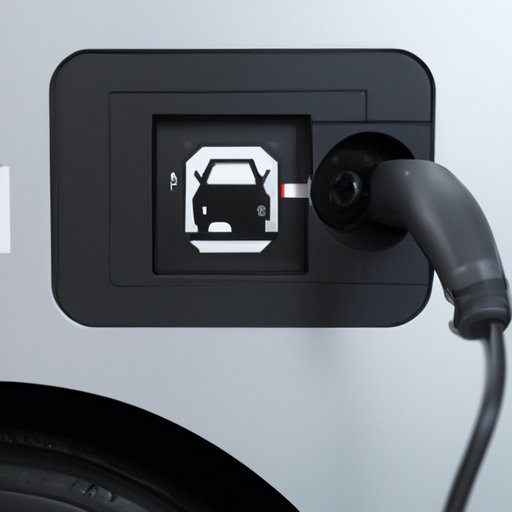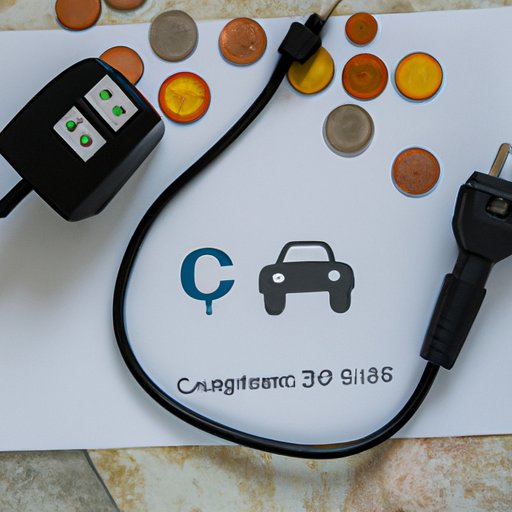# How Much Does it Cost to Charge an Electric Car at Home?## Introduction

Electric vehicles are becoming increasingly popular, as they offer a more sustainable means of transportation than traditional gasoline-powered cars. However, one of the most common questions asked by potential electric vehicle owners is “How much does it cost to charge an electric car at home?” This article will explore this question in detail, discussing the factors that influence the cost of home charging an electric car and offering tips on how to reduce these costs.

## Calculating the Cost of Home Charging an Electric Vehicle

The cost of home charging an electric vehicle depends on various factors, including local electricity rates, the size of the battery, and the amount of energy used to charge it. To accurately calculate the cost of home charging an electric vehicle, it is important to understand these factors and how they affect the cost.

### Understanding Electricity Rates

The cost of electricity is based on the rate charged by utility companies, which varies from region to region. The rate is usually expressed in cents per kilowatt hour (kWh). To calculate the cost of home charging an electric vehicle, you will need to know the local electricity rate, as well as the amount of energy used to charge the vehicle.

### Calculating Cost of Home Charging

Once you have determined the local electricity rate, you can then calculate the cost of charging your electric vehicle at home. To do this, multiply the local electricity rate by the amount of energy used to charge the vehicle. For example, if the local electricity rate is 10 cents per kWh and the vehicle uses 5 kWh to charge, the cost of home charging would be 50 cents.

## A Guide to Home Charging Your Electric Car: Know Your Costs

In order to accurately calculate the cost of home charging your electric car, there are two key pieces of information you will need to know: the amount of energy used to charge the vehicle (in kWh) and the number of miles it can travel on a single charge (in miles per kWh).

### Understanding kWh and Miles Per kWh

The amount of energy used to charge an electric vehicle is measured in kilowatt hours (kWh). A kWh is the amount of energy required to power a 1,000-watt appliance for one hour. The number of miles an electric vehicle can travel on a single charge is measured in miles per kWh. This is the number of miles the vehicle can go on a single kWh of energy.

### Estimating Total Cost of Home Charging

Once you have determined the local electricity rate and the amount of energy used to charge the vehicle, you can estimate the total cost of home charging. To do this, simply multiply the local electricity rate by the number of kWh used to charge the vehicle. For example, if the local electricity rate is 10 cents per kWh and the vehicle uses 5 kWh to charge, the total cost of home charging would be 50 cents.Understanding the True Cost of Charging an Electric Vehicle at Home

## Understanding the True Cost of Charging an Electric Vehicle at Home

In addition to the cost of home charging, there are other factors that can affect the total cost of owning and operating an electric vehicle. These include the cost of purchasing or leasing the vehicle, maintenance and repair costs, fuel costs, and taxes and fees.

### Factors Affecting Home Charging Cost

The cost of home charging an electric vehicle can vary depending on a number of factors, such as the type of charger being used, the local electricity rate, the size of the battery, and the amount of energy used to charge it. Additionally, some chargers may require additional installation costs, such as wiring, mounting, and other setup fees.

### Comparing Home Charging with Public Charging

It is also important to consider the cost of public charging when determining the total cost of owning and operating an electric vehicle. Public charging stations typically charge higher rates than home charging, so it is important to compare the cost of home charging with the cost of public charging before making a decision.

## How Much Does it Cost to Charge an Electric Car at Home?

The cost of home charging an electric car can vary depending on several factors, including the local electricity rate, the size of the battery, and the amount of energy used to charge it. In order to accurately calculate the cost of home charging an electric car, it is important to understand these factors and how they affect the cost.

### Factors Influencing Home Charging Cost

The cost of home charging an electric car can be affected by several factors, including the type of charger being used, the local electricity rate, the size of the battery, and the amount of energy used to charge it. Additionally, some chargers may require additional installation costs, such as wiring, mounting, and other setup fees.

### Checking Electricity Rates

To accurately calculate the cost of home charging an electric car, it is important to know the local electricity rate. This rate is usually expressed in cents per kilowatt hour (kWh). Checking the local electricity rate is the first step in determining the cost of home charging an electric car.

## What is the Cost of Home Charging an Electric Vehicle?

The cost of home charging an electric vehicle depends on various factors, including local electricity rates, the size of the battery, and the amount of energy used to charge it. To accurately calculate the cost of home charging an electric vehicle, it is important to understand these factors and how they affect the cost.

### Calculating Home Charging Cost

Once you have determined the local electricity rate and the amount of energy used to charge the vehicle, you can calculate the cost of home charging. To do this, simply multiply the local electricity rate by the number of kWh used to charge the vehicle. For example, if the local electricity rate is 10 cents per kWh and the vehicle uses 5 kWh to charge, the total cost of home charging would be 50 cents.

### Considering Battery Capacity

In addition to the local electricity rate, the size of the battery can also affect the cost of home charging an electric vehicle. Generally speaking, the larger the battery, the more energy it will require to charge, resulting in higher home charging costs. Therefore, it is important to consider the battery capacity when calculating the cost of home charging an electric vehicle.

## Budgeting for Home Charging: How Much Will It Cost to Charge an Electric Car?

When budgeting for home charging, it is important to calculate the cost of home charging an electric car accurately. To do this, you will need to know the local electricity rate, the size of the battery, and the amount of energy used to charge it. Additionally, there are a few tips that can help to reduce the cost of home charging an electric car.

### Calculating Cost of Home Charging

To calculate the cost of home charging an electric car, simply multiply the local electricity rate by the amount of energy used to charge the vehicle. For example, if the local electricity rate is 10 cents per kWh and the vehicle uses 5 kWh to charge, the total cost of home charging would be 50 cents.

### Tips for Reducing Home Charging Costs

There are a few tips that can help to reduce the cost of home charging an electric car. These include using a timer to ensure the car is only charging during off-peak hours, taking advantage of any available discounts or incentives, and installing a Level 2 charger, which is more efficient than a standard charger.

## Conclusion

Home charging an electric car can be a convenient and cost-effective way to keep your vehicle powered up. By understanding the factors that influence the cost of home charging, such as local electricity rates, battery capacity, and the amount of energy used to charge the vehicle, you can accurately estimate the total cost of home charging. Additionally, there are a few tips that can help to reduce the cost of home charging an electric car.#### By Happy Sharer

Hi, I'm Happy Sharer and I love sharing interesting and useful knowledge with others. I have a passion for learning and enjoy explaining complex concepts in a simple way.